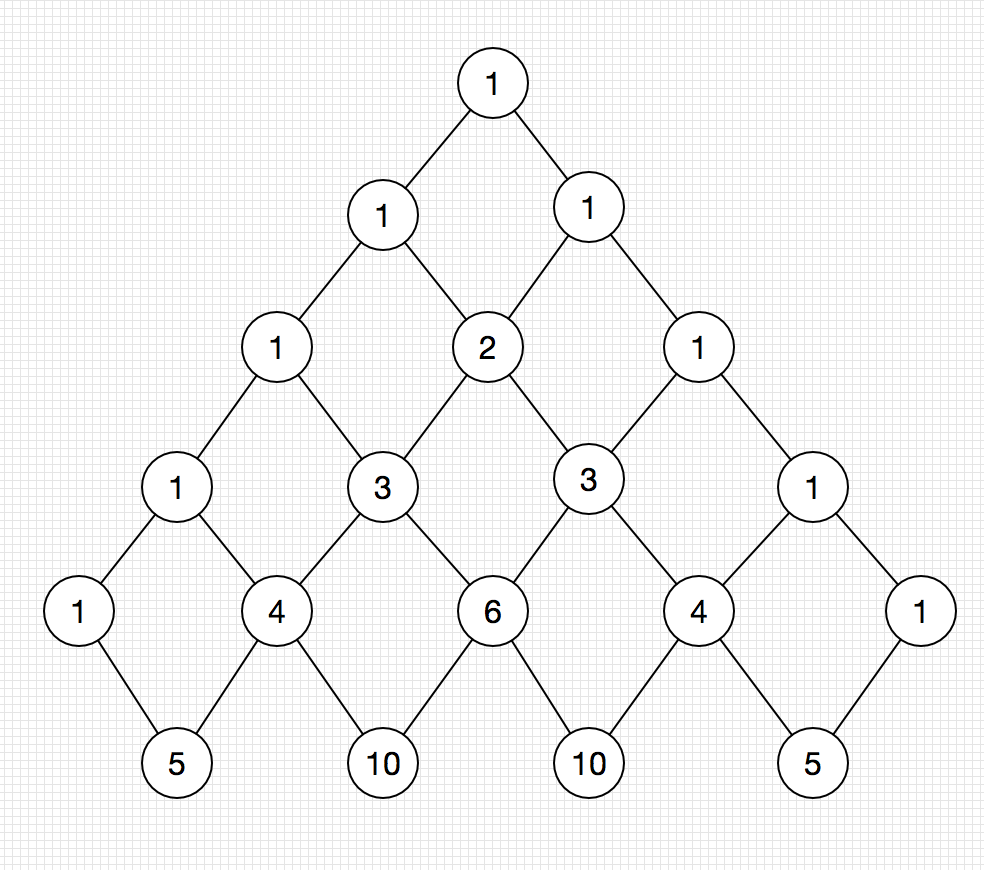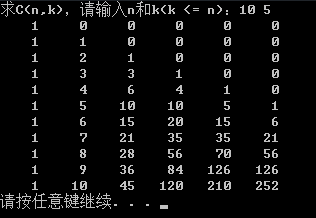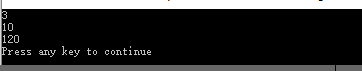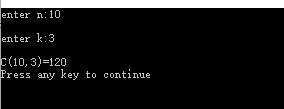• 动态规划之二项式系数@(算法学习)(nk)=n!(n−k)!k!{ n \choose k} = {n! \over {(n-k)!k!}} 计算二项式系数的问题在于，系数本身在int表示范围内，但是计算用到的分子是阶乘，这个是很大的数，会导致溢出的问题。...
动态规划之二项式系数

@(算法学习)

(nk)=n!(n−k)!k!<!--//--><![CDATA[//><!--
{ n \choose k} = {n! \over {(n-k)!k!}}
//--><!]]>

计算二项式系数的问题在于，系数本身在int表示范围内，但是计算用到的分子是阶乘，这个是很大的数，会导致溢出的问题。

所以，比较好的计算方法是运用帕斯卡三角形总结的规律求解。第一行表达的是：(00)=1<!--//--><![CDATA[//><!--
{0 \choose 0} = 1
//--><!]]>
第二行表达的是：(10)=1,(11)=1<!--//--><![CDATA[//><!--
{1 \choose 0} = 1, {1 \choose 1} = 1
//--><!]]>
第三行表达的是：(20)=1,(21)=2,(22)=1<!--//--><![CDATA[//><!--
{2 \choose 0} = 1, {2 \choose 1} = 2, {2 \choose 2} = 1
//--><!]]>

…

更有趣的是，每一个数是肩头两个数字之和。

运用的规律是：(nk)=(n−1k−1)+(n−1k)<!--//--><![CDATA[//><!--
{n \choose k} = {n - 1 \choose k - 1} + {n - 1 \choose k}
//--><!]]>，这个翻译成中文很好理解。从n个东西中选取k个，在面对第k件东西时，有一个决策，这件不选或者选两条路径。选了第k件则从剩下的n-1件里选k-1件即可。如果不选，就要从剩下的n-1件中选择k件。

这个思想在背包问题中运用的尤其广泛。背包问题是动态规划问题的一种模型，因此，也可以侧面反映动态规划的思想。

#include <stdio.h>

#define MAXN 100

long binomial_coefficient(int n, int m)// 从n中选择m
{
int i,j;
long bc[MAXN][MAXN]; //二项式系数表
for(i = 0; i <= n; i++) //帕斯卡三角每行第一个数全是1
{
bc[i] = 1;
}
for(j = 0; j <= n; j++) // 帕斯卡三角每行最后一个数全是1
{
bc[j][j] = 1;
}

for(i = 1; i <= n; i++) //状态转移方程
{
for(j = 1; j < i; j++)
{
bc[i][j] = bc[i-1][j-1]+bc[i-1][j];
}
}
return bc[n][m];
}
int main()
{
int n, m;
while(scanf("%d%d",&n,&m))
{
int res = binomial_coefficient(n,m);
printf("Result =  %d\n", res);
}

return 0;
}
展开全文动态规划
• 研究了python求二项式系数的几种方法，对比了一下他们的速度
最近研究了python求二项式系数的几种方法，对比了一下他们的速度
1. 利用阶乘简洁求
#普通阶乘
def fact(n):
if n == 0:
return 1
else:
return n*fact(n-1)
#普通Cmn
def Cmn(n,m):
return fact(n)/(fact(n-m)*fact(m))
2. 直接递归求解
def Cnk0(n,k):
if k==0: return 1
if n==0: return 0
return Cnk0(n-1,k)+Cnk0(n-1,k-1)

3. 动态规划求解
（1）带备忘录的
def memo(func):
cache={}
@wraps(func)
def wrap(*args):
if args not in cache:
cache[args]=func(*args)
return cache[args]
return wrap
@memo
def Cnk(n,k):
if k==0: return 1
if n==0: return 0
return Cnk(n-1,k)+Cnk(n-1,k-1)
（2）迭代求解
def CnkD(n,k):
C=defaultdict(int)
for row in range(n+1):
C[row,0]=1
for col in range(1,k+1):
if col <= row:
C[row,col]=C[row-1,col-1]+C[row-1,col]
return C[n,k]
速度对比：

n = 100
k = 5
用时（秒）：
1： 0.0
2：64.45168709754944
3（1）：0.0019998550415039062
3（2）：0.0
发现直接递归求解的速度是最慢的，这个容易想见，因为其做了很多重复运算
去掉它，再进一步对比剩下三个的速度

n = 100
k = 80
用时（秒）：1： 0.03（1）：0.0109999179840087893（2）：0.007001161575317383

n = 500
k = 100
这时发现1，和3（1）都出现了maximum recursion depth exceeded in comparison错误

这是由于栈深度引起的问题
只有3（2）不存在这个问题仍能计算出结果


展开全文python 性能
• 由于子问题的交叠性质，所以采用递归地方法一次又一次地求解子问题时，进行了很多重复的工作。所以动态规划法建议：把子问题的解存入某个表中，通过表一步步反解出原始问题。斐波那契数列就是一个很好的例子： F(n) ...
如果问题是由交叠的子问题所构成的，那么我们就可以用动态规划技术来解决它。也就是说，一个问题的解可由它的规模更小的子问题的解递推得出。由于子问题的交叠性质，所以采用递归地方法一次又一次地求解子问题时，进行了很多重复的工作。所以动态规划法建议：把子问题的解存入某个表中，通过表一步步反解出原始问题。斐波那契数列就是一个很好的例子：
F(n) = F(n-1) + F(n-2)     当n≥2
F(0) = 0
F(1) = 1
如果直接采用递推公式求解第n个斐波那契数，那么会重复大量的无用工作，效率变得极为低下。同时注意到，F(n-1)和F(n-2)是两个规模更小的具有交叠性质的子问题，所以可以使用动态规划法求解F(n)。方法是创建一个表保存每一个斐波那契数。这使得每个斐波那契数只求解了一次，求解F(n)只需要查表获得F(n-1)和F(n-2)的值即可。

动态规划其实和一般的递归方法很相似，核心在于求出一个递推式和一个边界条件。不同的是，动态规划会保存中间结果并利用这些中间结果解出最终的问题。

下面一个例子是利用动态规划计算二项式系数。

给出n和k，求出二项式系数C(n,k)。在这里，我们只关心两个信息就够了：

C(n,k) = C(n-1,k-1) + C(n-1,k)    当n>k>0C(n,0) = C(n,n) = 1从上面的递推式可以看到两个较小的具有交叠性质的子问题C(n-1,k-1)和C(n-1,k)，所以，计算二项式系数是把动态规划应用于非最优化问题的一个标准例子。

废话不多说，直接上代码：

#include <iomanip>
#include <iostream>

using namespace std;

int main()
{
int n, k;

cout << "求C(n,k)，请输入n和k(k <= n)：";
cin >> n >> k;

int **p;
p = new int*[n + 1];
for (int i = 0; i <= n; i++)
p[i] = new int[k + 1];

for (int i = 0; i <= n; i++)
for (int j = 0; j <= k; j++)
p[i][j] = 0;

for (int i = 0; i <= n; i++)
p[i] = 1;  // C(n,0) = 0

for (int i = 0; i <= k; i++)
p[i][i] = 1;  // C(n,n) = 1

for (int i = 2; i <= n; i++)
for (int j = 1; j < (i > k ? k + 1 : i); j++)
p[i][j] = p[i - 1][j - 1] + p[i - 1][j];

//cout.flags(ios::right);   // 输出对齐
for (int i = 0; i <= n; i++)
{
for (int j = 0; j <= k; j++)
cout << setw(5) << p[i][j] << ' ';

cout << endl;
}

// 销毁空间
for (int i = 0; i <= n; i++)
delete[] p[i];

delete[] p;

return 0;
}
运行结果：矩阵右下角便是所要求的结果。可以看到，这个矩阵正是杨辉三角，即帕斯卡三角的一部分。

算法效率：
该算法的核心操作是加法，时间复杂度为Θ(nk)。

参考：
《算法设计与分析基础》8.1节。


展开全文• 对于学习C语言的一般都知道我们需要练习使用程序求二项式系数。今天我主要给大家分享使用迭代、递归、动态规划求二项式系数，同时分析算法时间空间复杂性。对于迭代和递归的概念，我之前也有讲解，现在呢？给大家...
对于学习C语言的一般都知道我们需要练习使用程序求二项式系数。今天我主要给大家分享使用迭代、递归、动态规划求二项式系数，同时分析算法时间空间复杂性。对于迭代和递归的概念，我之前也有讲解，现在呢？给大家讲解一下动态规划的概念。动态规划是五大常用的算法之一，动态规划过程是：每次决策依赖于当前状态。又随即引起状态的转移。一个决策序列就是在变化的状态中产生出来的，所以，这样的多阶段最优化决策解决这个问题的过程就称为动态规划。 动态规划是运筹学中用于求解决策过程中的最优化数学方法 。通常假设问题是由交叠的子问题所构成，我们就能够用动态规划技术来解决它。一般来说，这种子问题出如今对给定问题求解的递推关系中，这个递推关系包括了同样问题的更小子问题的解。动态规划法建议，与其对交叠子问题一次重新的求解，不如把每一个较小子问题仅仅求解一次并把结果记录在表中（动态规划也是空间换时间的）。这样就能够从表中得到原始问题的解。动态规划经常常使用于解决最优化问题。完整代码如下。迭代程序源代码:#include<iostream>
using namespace std;
int C(int n,int m)
{
if(m==0 || m==n) return 1;
else return C(n-1,m-1)+C(n-1,m);//迭代算法控制
}
int main()
{
int n,m;
cin>>n>>m;
cout<<C(n,m)<<endl;
return 0;
}结果：分析：迭代是重复反馈过程的活动，其目的通常是为了逼近所需目标或结果。每一次对过程的重复称为一次"迭代"，而每一次迭代得到的结果会作为下一次迭代的初始值。所以时间复杂度应该就是O(C(n,m)),空间复杂度应该为O(n)。递归算法计算二项式系数程序代码：#include<stdio.h>
int f(int n,int k)
{
if(k==0||k==n) //出口
return 1;
else
return f(n-1,k)+f(n-1,k-1);//阶乘
}
void main(){
int n,k,c;
scanf("%d%d",&k,&n);
c=f(n,k);
printf("%d\n",c);
}结果:分析：递归就是在运行的过程中调用自己。使用递归解决问题，思路清晰，代码少。但是使用递归算法要耗用更多的栈空间，所以在堆栈尺寸受限制时（如嵌入式系统或者内核态编程。所以该算法的时间复杂度为O（n^2）,空间复杂度为O（n）。动态规划算法计算二项式系数程序代码：#include <iostream>
#include <cstdlib>
using namespace  std;
long Binomial(int,int);
int main()
{
int n,k;
cout<<"输入n:";
cin>>n;
cout<<"\输入k:";
cin>>k;
cout<<"\nC("<<n<<","<<k<<")="<<Binomial(n,k)<<endl;
return 0;
}
int min(int n,int m)
{
return (n>m)?m:n;
}
long Binomial(int n,int k)
{
if (n<k||n<0||k<0)
{
cout<<"数据为：\n";
return -1;
}
else
{
int** a=new int*[n+1];
if (a==0)
{
cout<<"不能分配\n";
return -2;
}
int i,j;
for (i=0;i<=n;i++)
{
a[i]=new int[k+1];
if (a[i]==0)
{
cout<<"不能分配\n";
return -3;
}
}
for (i=0;i<=n;i++)
{
for (j=0;j<=min(i,k);j++)
if (j==0||j==i)
a[i][j]=1;
else
a[i][j]=a[i-1][j]+a[i-1][j-1];
}
int temp=a[n][k];
for (i=0;i<=n;i++)
delete [] a[i];
delete [] a;
return temp;
}
}结果：分析：用动态规划解题的好处，高效、算法清晰简便、程序易编、易调。但是运用动态规划时，必须对具体问题进行具体的分析处理，需要丰富的想象力去建立数学模型，需要创造性的思想去解决问题。动态态规划需要按阶段遍历整个状态空间空间。从分析中，我们可以看出，我们是从已知最优值的初始状态和边界状态出发，利用最优化原理，一步一步向未知目标状态推进，直到目标状态的最优值解决。
展开全文递归 迭代 动态规划
• 例如：计算 C( n , k ) ,  代码如下：   function y = Binomial( n , k ) %UNTITLED Summary of this function goes here % Detailed explanation goes here c = [ ] ;  for i = 1 : n
• 在上一篇文章中，我总结了从阅读《编程珠玑I》中获得的一些启示。... 作为代码重审和回顾的一个例子，我对以前的一个粗糙的二项式定理实现进行了重审和改写。当时，主要是为了学习动态规划法技术，运Java 代码改进 动态规划
• 知识点：伯努利分布、二项式分布、多项式分布、先验概率，后验概率，共轭分布、贝塔分布、贝塔-二项分布、负二项分布、狄里克雷分布，伽马函数、分布 一，伯努利分布(bernouli distribution) 又叫做0-1分布，...
• 在高中的时候，我们一般用组合数求二项式展开式系数，但是对于编程，这一方法就显得十分勉强。因为当n较大时，n的阶乘非常大，很难有数据类型能够存储。虽然用杨辉三角的方法也会出现类似情况，但是杨辉三角c语言
• 他们都可以看着是参数分布，因为他们的函数形式都被一小部分的参数控制，比如正态分布的均值和方差，二项式分布事件发生的概率等。因此，给定一堆观测数据集（假定数据满足独立同分布），我们需要有一个解决方案来...
• 给定aaa求是否有xxx满足这个子，若有r则称a是模p的次剩余 若没有满足条件的xxx，则称a是模p的非次剩余 然而在一些题目中，我们既要判定它是否是模p的次剩余，也要判断其值，本文就对此进行一些探究 对于所有...
• 行列计算 前言 一、对角线法 、代数余子式法 三、等价转化法 四、逆序数法 总结 本文主要讲述行列的求解方法，所以本文侧重于方法的讲解，而并非推导。主要思路为从三阶行列举例，再过渡到高阶行列的...线性代数 矩阵
• 原文转自：... ...对于典型的离散型随机变量分布：二项式分布，多项式分布；...他们都可以看着是参数分布，因为他们的函数形式都被一小部分的参数控制，比如正态分布的均值和方差，二项式分布事件发生的概
• ## 计算方法知识总结

千次阅读 2016-12-23 18:36:05
• 单样本二项式检验（binomial test） 问题：调查北京市所有人喜欢吃面食还是吃米饭（都不喜欢吃的忽略），在北京街头随机选了10个人（样本有点少），有8个喜欢吃面食，2个喜欢吃米饭。由此能否否定北京人喜欢吃面食的...统计检验
• ## 因子和的计算方法

千次阅读 2015-06-28 10:45:22
因子和的计算方法 神马叫因子和：一个数的所以因子的和就叫因子和。。。 ...证明写起来比较麻烦，大体上思路就是牛顿二项式。。。 例如： 12 = 2 * 2 * 3 12的因子为1 2 3 4 6 12
• 之前一网友遇到类似问题，特查了相关文献，归结一下方法。 1.根据各方法计算公式进行编辑公式，data 步就可以搞定。相关文献有：《A Comparison of Binomial Proportion Interval Estimation Methods 》、...intervals parameters macros statistics
• ## 二项分布

千次阅读 2013-12-11 09:58:48
二项分布[编辑] 维基百科，自由的百科全书 二项分布 机率 质量 函数 累积分布函数 参数  试验次数 (整数)  成功概率 (实数) 值域 概率密度函数 ...维基百科
• 转自：大数量级组合数的快速计算方法 由下面的组合数公式可以推导 为了解决第个效率的问题，我们对上再做一步化简。上已经把连乘法变成了求和的线性运算，也就是说，上已经极大地简化了计算的复杂度，...组合数 数学
• Modularity，中文称为模块度，是 Community Detection（社区发现/社团检测） 中用来衡量社区划分质量的一种方法。要理解Modularity，我们先来看社团和社团检测的概念。社团检测社团检测，就是要在一个图（包含顶点...
•  项式（例如：1010 0000 0000 0001）进行异或； （5）、重复步骤3和4，直到右移8次，这样整个8位数据全部进行了处理； （6）、重复步骤2到步骤5，进行通讯信息帧下一个字节的处理； （7）、将该通讯信息帧...CRC
• 词语的语义相似度计算主要有两种方法 ：一类是通过语义词典，把有关词语的概念组织在一个树形的结构中来计算；另一类主要是通过词语上下文的信息，运用统计的方法进行求解。 1. 语义相似度Dekang Lin认为任何两个...
• ## 1.4 行列式计算

千次阅读 2020-01-07 14:52:14
文章目录纯数字类型的行列·例1·例2元素相同位置不同的行列·例3加边法三叉型（鸡爪型）行列范德蒙行列反对称行列对称行列参考 纯数字类型的行列 ·例1 技巧： 化成上三角计算 计算之前对行进行...
• 概述 在信息爆炸时代，人们迫切...因此了解文本相似度的计算方法是很有必要的。 文本相似度定义 文本相似度在不同领域被广泛讨论，由于应用场景不同，其内涵有所差异，故没有统一、公认的定义。 Lin从信息论的角度...文本相似度 word2vec simhash 神经网路 搜索引擎
• RAID磁盘阵列组介绍（常用） 磁盘阵列（RedundantArrays ofIndependentDisks，RAID），有“独立磁盘构成的具有...利用这技术，将数据切割成许多区段，分别存放在各个硬盘上。 磁盘阵列还能利用同位检查（Parity C...
• ## CRC计算方法与实例

万次阅读 2015-03-24 23:44:23
CRC计算方法与实例   文章编号： 090107122214 文章分类： EDA技术 ＞ Keil C   点 击： ... ...
• ## KDJ指标的计算方法

千次阅读 2009-06-17 21:56:17
指标KDJ的计算比较复杂，首先要计算周期（n日、n周等）的RSV值，即未成熟随机指标值，然后再计算K值、D值、J值等。以日KDJ数值的计算为例，其计算公式为 n日RSV=（Cn－Ln）÷（Hn－Ln）×100中，Cn为第n日收盘价...
•算法 java
• 营改增后土地增值税计算方法为：应纳土地增值税=增值额×税率...